##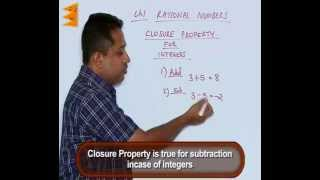By Escholarindia Faridabad# ADDITION & SUBTRACTION OF RATIONAL EXPRESSIONS WITH DIFFERENT DENOMINATORS (ANIMATION)

##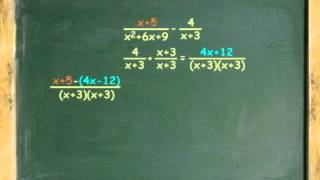By Jerry Llevada

ADDITION & SUBTRACTION OF RATIONAL EXPRESSIONS WITH DIFFERENT DENOMINATORS (ANIMATION)# Finding Products of Polynomials

##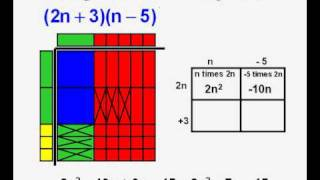By gdawgrapper

Finding Products of Polynomials# Example 3: Multiplying a monomial by a polynomial | Algebra I | Khan Academy

##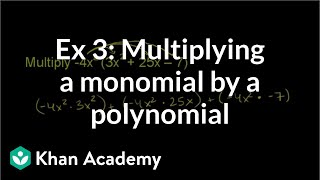By Khan Academy

Example 3: Multiplying a monomial by a polynomial | Algebra I | Khan Academy# The Remainder Theorem - Example 1

##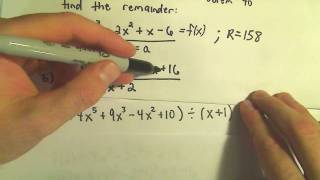By PatrickJMT

Youtube Presents The Remainder Theorem - Example 1 an educational video resources on english language arts.# Polynomials - Introduction of Identities - YouTube

##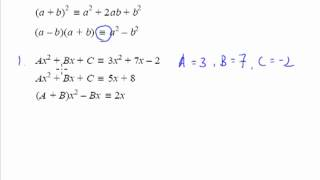By numberskill Math Tuition

Proving polynomial identities# Binomial theorem combinatorics connection | Algebra II | Khan Academy

##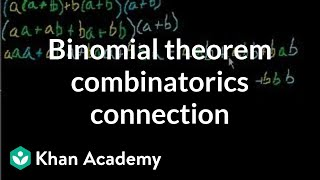By Khan Academy

Intuition behind why binomial expansion involves combinatorics.# Exploring the Binomial Theorem

##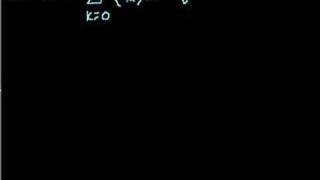By Khan Academy

Watch this video which introduced the Bionomial Theorem--a way to expand (a + b)n where n is any positive integer and x and y are any numbers.# Multiplying and dividing rational expressions 2 | Algebra II | Khan Academy

##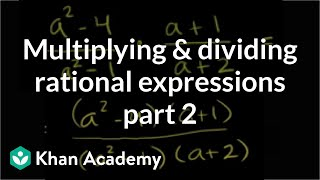By Khan Academy

Khan Academy presents Multiplying and Dividing Rational Expressions 2, an educational video resource on math.##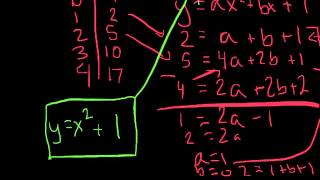By MMRHS Math Help

From a table, writing relationships in explicit and recursive form# Applying rational equations 2 | Polynomial and rational functions | Algebra II | Khan Academy

##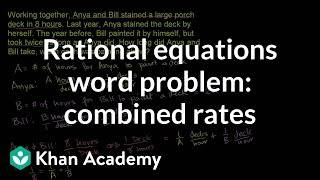By Khan Academy

Khan Academy presents Applying Rational Equations 2, an educational video resource on math.# Khan Academy: Introduction to Functions

##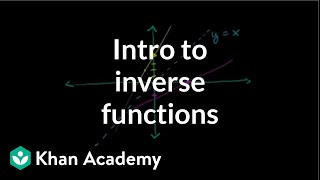By Khan Academy

This video lecture series on Algebra from Khan Academy includes Linear Equations, Solving Inequalities, Slope and Y-intercept, Equation of a Line, Averages, Integer sums, Taking Percentages, Systems of Equations, Ratios, Multiplying Expressions, Solving a quadratic by factoring, Imaginary Numbers, Complex Numbers, Quadratic Equations, Quadratic Inequalities, Functions, Conic sections, Circles, Ellipses, Hyperbolas..##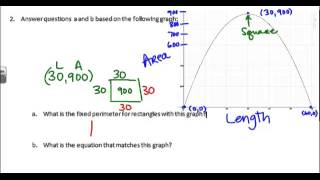By Megan Frantz# Completing the square for vertex form | Quadratic equations | Algebra I | Khan Academy

##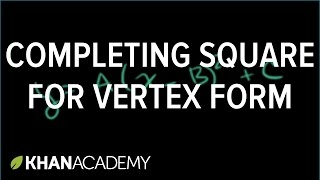By Khan Academy

An example problem on how to complete the square to write a quadratic function in vertex form.##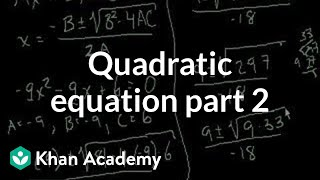By Khan Academy

This video lecture series on Algebra from Khan Academy includes Linear Equations, Solving Inequalities, Slope and Y-intercept, Equation of a Line, Averages, Integer sums, Taking Percentages, Systems of Equations, Ratios, Multiplying Expressions, Solving a quadratic by factoring, Imaginary Numbers, Complex Numbers, Quadratic Equations, Quadratic Inequalities, Functions, Conic sections, Circles, Ellipses, Hyperbolas##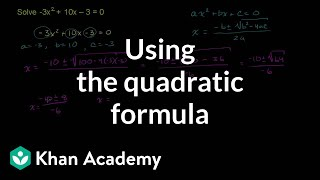By Khan Academy# Geometric Means in a Geometric Sequence

##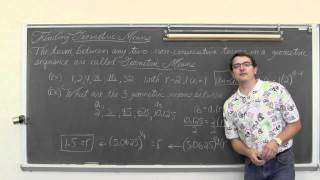By ProfRobBob

This video lesson explains how to find missing geometric means within a geometric sequence. Two example problems are included# Finding the Inverse of a Function or Showing One Does not Exist, Ex 3

##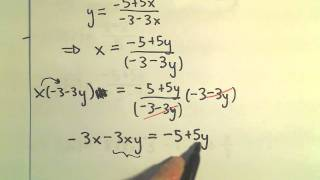By PatrickJMT

YouTube presents Finding the Inverse of a Function or Showing One Does not Exist, Ex 3, an educational video resource on math.# The Intercept Method - MathHelp.com - Algebra Help

##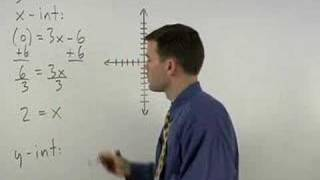By yourteachermathhelp

YouTube presents The Intercept Method, an educational video resource on math.# Visualizing Taylor Series for e^x

##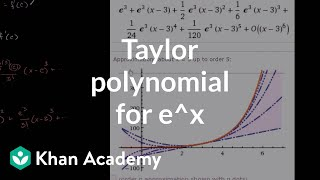By Khan Academy

Visualizing Taylor Series for e^x